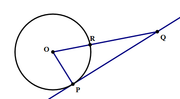## In the diagram above, line $$PQ$$ is tangent to the circle,

##### This topic has expert replies
Moderator
Posts: 1610
Joined: 29 Oct 2017
Thanked: 1 times
Followed by:5 members

### In the diagram above, line $$PQ$$ is tangent to the circle,

by M7MBA » Fri Dec 13, 2019 11:11 amIn the diagram above, line $$PQ$$ is tangent to the circle, and the measure of arc $$PR$$ is $$70Â°.$$ What is the measure of $$âˆ PQR?$$

A. $$15Â°$$
B. $$20Â°$$
C. $$25Â°$$
D. $$30Â°$$
E. $$35Â°$$

[spoiler]OA=B[/spoiler]

Source: Magoosh

Newbie | Next Rank: 10 Posts
Posts: 8
Joined: 12 Dec 2019
by dsan6422 » Mon Dec 16, 2019 6:04 pm

Junior | Next Rank: 30 Posts
Posts: 11
Joined: 30 Nov 2019
by Pyunika » Tue Dec 17, 2019 10:52 am
angle p makes 90 degree since it is the tangent to the circle.
angle r is given as 70 degree
and sum of all the angles in the triangle should be 180. thus angle pqr equals 20 degree

### GMAT/MBA Expert

GMAT Instructor
Posts: 15463
Joined: 08 Dec 2008
Location: Vancouver, BC
Thanked: 5254 times
Followed by:1266 members
GMAT Score:770
by [email protected] » Wed Dec 18, 2019 6:20 am
M7MBA wrote:In the diagram above, line $$PQ$$ is tangent to the circle, and the measure of arc $$PR$$ is $$70Â°.$$ What is the measure of $$âˆ PQR?$$

A. $$15Â°$$
B. $$20Â°$$
C. $$25Â°$$
D. $$30Â°$$
E. $$35Â°$$
There's a real nice Circle Property rule that says: A line tangent to a circle will be perpendicular to the line passing through the center and the point of tangency
This means âˆ OPQ = 90Â°
We are also told that âˆ OPR = 70Â°

Since all three angles in a triangle must add to 180Â°, we can conclude that âˆ PQR = 20Â°

Cheers,
Brent

### GMAT/MBA Expert

GMAT Instructor
Posts: 6195
Joined: 25 Apr 2015
Location: Los Angeles, CA
Thanked: 43 times
Followed by:24 members
by [email protected] » Wed Jan 01, 2020 6:43 pm
M7MBA wrote:In the diagram above, line $$PQ$$ is tangent to the circle, and the measure of arc $$PR$$ is $$70Â°.$$ What is the measure of $$âˆ PQR?$$

A. $$15Â°$$
B. $$20Â°$$
C. $$25Â°$$
D. $$30Â°$$
E. $$35Â°$$

[spoiler]OA=B[/spoiler]

Source: Magoosh
While we are not told that it is so, we assume that O is the center because otherwise, the answer cannot be determined from the given information.

Since arc PR = 70 degrees, then the intercepted central angle (angle ROP) is also 70 degrees. Furthermore, since line PQ is tangent to the circle, angle OPQ = 90 degrees. Therefore, angle PQR = 180 - 70 - 90 = 20 degrees.